Home
Hostname: page-component-8bbf57454-nshs2 Total loading time: 0.23 Render date: 2022-01-26T08:18:16.324Z Has data issue: true Feature Flags: { "shouldUseShareProductTool": true, "shouldUseHypothesis": true, "isUnsiloEnabled": true, "metricsAbstractViews": false, "figures": true, "newCiteModal": false, "newCitedByModal": true, "newEcommerce": true, "newUsageEvents": true }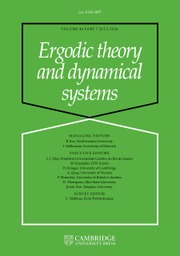Ergodic Theory and Dynamical Systems

A consequence of the growth of rotation sets for families of diffeomorphisms of the torus

Published online by Cambridge University Press:  04 December 2018

Abstract

In this paper we consider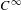$C^{\infty }$-generic families of area-preserving diffeomorphisms of the torus homotopic to the identity and their rotation sets. Let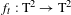$f_{t}:\text{T}^{2}\rightarrow \text{T}^{2}$ be such a family,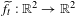$\widetilde{f}_{t}:\mathbb{R}^{2}\rightarrow \mathbb{R}^{2}$ be a fixed family of lifts and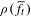$\unicode[STIX]{x1D70C}(\widetilde{f}_{t})$ be their rotation sets, which we assume to have interior for$t$ in a certain open interval$I$. We also assume that some rational point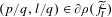$(p/q,l/q)\in \unicode[STIX]{x2202}\unicode[STIX]{x1D70C}(\widetilde{f}_{\overline{t}})$ for a certain parameter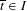$\overline{t}\in I$, and we want to understand the consequences of the following hypothesis: for all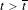$t>\overline{t}$,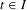$t\in I$,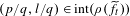$(p/q,l/q)\in \text{int}(\unicode[STIX]{x1D70C}(\widetilde{f}_{t}))$. Under these very natural assumptions, we prove that there exists a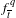$f_{\overline{t}}^{q}$-fixed hyperbolic saddle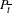$P_{\overline{t}}$ such that its rotation vector is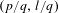$(p/q,l/q)$. We also prove that there exists a sequence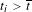$t_{i}>\overline{t}$,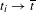$t_{i}\rightarrow \overline{t}$, such that if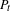$P_{t}$ is the continuation of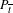$P_{\overline{t}}$ with the parameter, then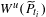$W^{u}(\widetilde{P}_{t_{i}})$ (the unstable manifold) has quadratic tangencies with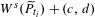$W^{s}(\widetilde{P}_{t_{i}})+(c,d)$ (the stable manifold translated by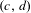$(c,d)$), where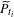$\widetilde{P}_{t_{i}}$ is any lift of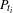$P_{t_{i}}$ to the plane. In other words,$\widetilde{P}_{t_{i}}$ is a fixed point for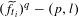$(\widetilde{f}_{t_{i}})^{q}-(p,l)$, and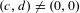$(c,d)\neq (0,0)$ are certain integer vectors such that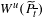$W^{u}(\widetilde{P}_{\overline{t}})$ do not intersect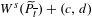$W^{s}(\widetilde{P}_{\overline{t}})+(c,d)$, and these tangencies become transverse as$t$ increases. We also prove that, for$t>\overline{t}$,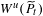$W^{u}(\widetilde{P}_{t})$ has transverse intersections with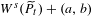$W^{s}(\widetilde{P}_{t})+(a,b)$, for all integer vectors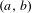$(a,b)$, and thus one may consider that the tangencies above are associated to the birth of the heteroclinic intersections in the plane that do not exist for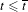$t\leq \overline{t}$.

MSC classification

Type
Original Article
Information
Ergodic Theory and Dynamical Systems , June 2020 , pp. 1441 - 1458

Access options

Get access to the full version of this content by using one of the access options below. (Log in options will check for institutional or personal access. Content may require purchase if you do not have access.)

References

Addas-Zanata, S.. Area-preserving diffeomorphisms of the torus whose rotation sets have non-empty interiors. Ergod. Th. & Dynam. Sys. 1 (2015), 133.Google Scholar
Addas-Zanata, S.. Uniform bounds for diffeomorphisms of the torus and a conjecture of Boyland. J. Lond. Math. Soc. (2) 91 (2015), 537553.Google Scholar
Addas-Zanata, S. and Le Calvez, P.. Rational mode locking for homeomorphisms of the 2-torus. Proc. Amer. Math. Soc. 146 (2018), 15511570.Google Scholar
Boyland, P., de Carvalho, A. and Hall, T.. New rotation sets in a family of torus homeomorphisms. Invent. Math. 204 (2016), 895937.Google Scholar
Bronzi, M. and Tahzibi, A.. Homoclinic tangency and variation of entropy. Cadernos de Matematica 10 (2009), 133143.Google Scholar
Brown, M.. A new proof of Brouwer’s lemma on translation arcs. Houston J. Math. 10 (1984), 3541.Google Scholar
Cartwright, M. and Littlewood, J.. Some fixed point theorems. Ann. of Math. (2) 54 (1951), 137.Google Scholar
Churchill, R. and Rod, D.. Pathology in dynamical systems III, analytic Hamiltonians. J. Differential Equations 37 (1980), 351373.Google Scholar
Duarte, P.. Elliptic isles in families of area preserving maps. Ergod. Th. & Dynam. Sys. 28 (2008), 17811813.Google Scholar
Dumortier, F. and Melo, W.. A type of moduli for saddle connections of planar diffeomorphisms. J. Differential Equations 75 (1988), 88102.Google Scholar
Dumortier, F., Rodrigues, P. and Roussarie, R.. Germs of Diffeomorphisms in the Plane (Lecture Notes in Mathematics, 902). Springer, Berlin, 1981.Google Scholar
Franks, J.. Realizing rotation vectors for torus homeomorphisms. Trans. Amer. Math. Soc. 311 (1989), 107115.Google Scholar
Franks, J. and Le Calvez, P.. Regions of instability for non-twist maps. Ergod. Th. & Dynam. Sys. 23 (2003), 111141.Google Scholar
Koropecki, A., Le Calvez, P. and Nassiri, M.. Prime ends rotation numbers and periodic points. Duke Math. J. 164 (2015), 403472.Google Scholar
Koropecki, A., Le Calvez, P. and Nassiri, M.. Prime ends and boundary dynamics. 2018, in preparation.Google Scholar
Koropecki, A. and Tal, F.. Strictly toral dynamics. Invent. Math. 196 (2014), 339381.Google Scholar
Kwapisz, J.. Rotation sets and entropy. PhD Thesis, SUNY at Stony Brook, 1995.Google Scholar
Mather, J.. Invariant subsets of area-preserving homeomorphisms of surfaces. Adv. Math. Suppl. Studies 7B (1994), 331351.Google Scholar
Meyer, K.. Generic bifurcation of periodic points. Trans. Amer. Math. Soc. 149 (1970), 95107.Google Scholar
Misiurewicz, M. and Ziemian, K.. Rotation sets for maps of tori. J. Lond. Math. Soc. 40 (1989), 490506.Google Scholar
Newhouse, S., Palis, J. and Takens, F.. Bifurcations and stability of families of diffeomorphisms. Publ. Math. Inst. Hautes Études Sci. 57 (1983), 571.Google Scholar
Oliveira, F.. On the generic existence of homoclinic points. Ergod. Th. & Dynam. Sys. 7 (1987), 567595.Google Scholar
Pujals, E. and Sambarino, M.. On homoclinic tangencies, hyperbolicity, creation of homoclinic orbits and variation of entropy. Nonlinearity 13 (2000), 921926.Google Scholar
Yan, J.. Existence of periodic points near an isolated fixed point with Lefschetz index 1 and zero rotation for area preserving surface homeomorphisms. Preprint, 2018.Google Scholar

Send article to Kindle

Note you can select to send to either the @free.kindle.com or @kindle.com variations. ‘@free.kindle.com’ emails are free but can only be sent to your device when it is connected to wi-fi. ‘@kindle.com’ emails can be delivered even when you are not connected to wi-fi, but note that service fees apply.

Find out more about the Kindle Personal Document Service.

A consequence of the growth of rotation sets for families of diffeomorphisms of the torus
Available formats
×

Send article to Dropbox

To send this article to your Dropbox account, please select one or more formats and confirm that you agree to abide by our usage policies. If this is the first time you use this feature, you will be asked to authorise Cambridge Core to connect with your <service> account. Find out more about sending content to Dropbox.

A consequence of the growth of rotation sets for families of diffeomorphisms of the torus
Available formats
×

To send this article to your Google Drive account, please select one or more formats and confirm that you agree to abide by our usage policies. If this is the first time you use this feature, you will be asked to authorise Cambridge Core to connect with your <service> account. Find out more about sending content to Google Drive.

A consequence of the growth of rotation sets for families of diffeomorphisms of the torus
Available formats
×
×